# Answers to Chapter 08 & 09 Study Questions

•• Contributed by Delmar Larsen
• Founder and Director at Libretexts

1. 6
2. 1
3. 3 (two s electrons and one p electron)

1. in order of increasing electronegativity: $$\mathrm{Ge < C < O}$$

1. $$\ce{Li+}$$
2. $$\ce{Na+}$$
3. $$\ce{F}$$

1. $$\ce{O^2-}$$, $$\ce{F-}$$, $$\ce{Na+}$$, $$\ce{Mg^2+}$$, $$\ce{Al^3+}$$
2. $$\ce{S^2-}$$, $$\ce{Cl-}$$, $$\ce{K+}$$, $$\ce{Ca^2+}$$, $$\ce{Sc^3+}$$
3. $$\ce{I-}$$, $$\ce{Cs+}$$, $$\ce{Ba^2+}$$

1. isoelectronic

1.2.3.4.5.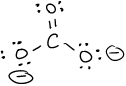6.7. $$\ce{P2Cl2}$$8. $$\ce{N2O4}$$9.10.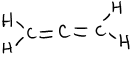or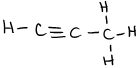1. bent, polar
2. linear, nonpolar
3. trigonal pyramid, polar
4. tetrahedral, polar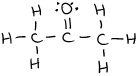or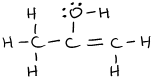1. Covalent bonds involve the sharing of electrons between two atoms (usually nonmetals). The orbitals of bonding electrons overlap so that the bonding electrons spend more time between the 2 atoms. A bond is polar when one of the atoms is more electronegative than the other atom. A molecule is polar when the overall molecule has a net dipole moment, that is, electrons spend more time on one side of the molecule than the other.

1. Chemical properties are shared within a Group but not within a Period. Group number is a good predictor of chemical properties; Period number is not.

1. $$\mathrm{C - F}$$
2. $$\mathrm{Si - F}$$

1. see-saw
2. square planar
3. trigonal bipyramid
4. octahedral

1. sp3
2. sp
3. sp3d2
4. sp3d
5. sp2
6. sp2

1. 4 sigma bonds
2. 3 sigma bonds, 1 pi bond
3. 2 sigma bonds, 2 pi bonds
4. 9 sigma bonds, 1 pi bond